# 3D Shape Properties Worksheet

📆 1 Dec 2022
🔖 Shape Category
📂 Gallery Type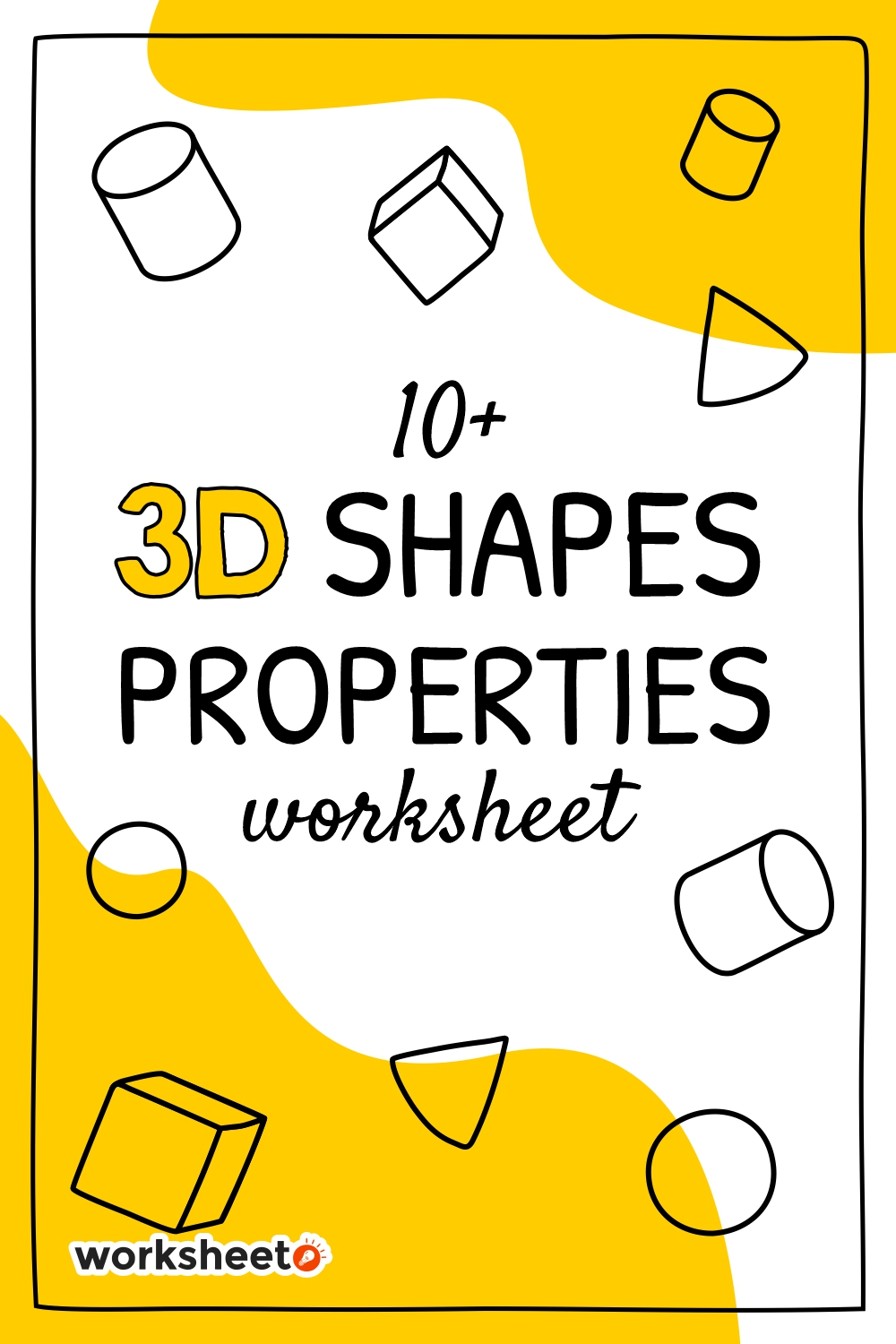9 Images of 3D Shape Properties Worksheet

3D Shape Properties Worksheet are designed to help students learn more about the properties of shapes. The worksheet includes 16 shape properties questions, and students can check the corresponding answer on the right side of the worksheet. The worksheet can be used to help reinforce concepts learned in class, or as a test preparation tool.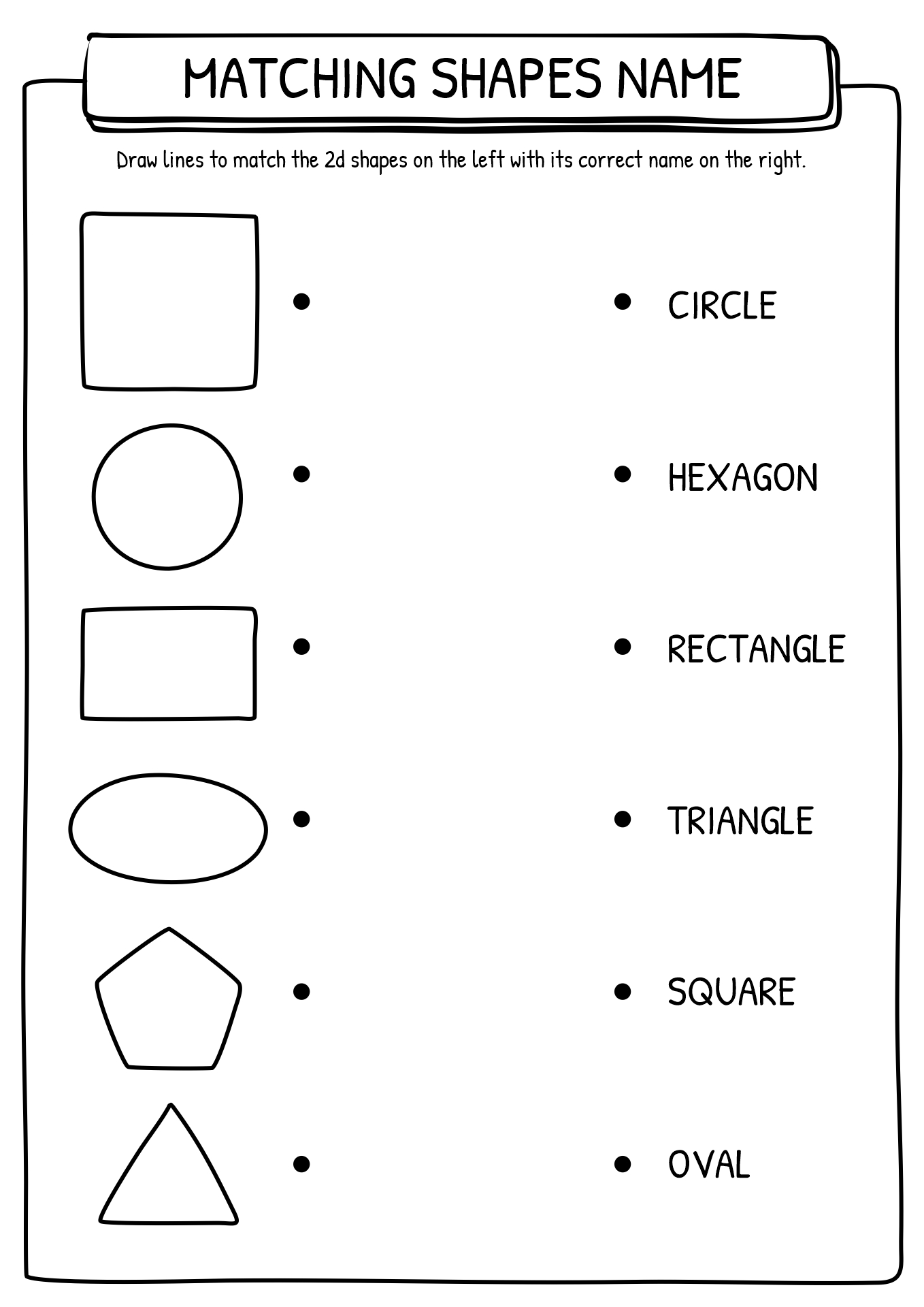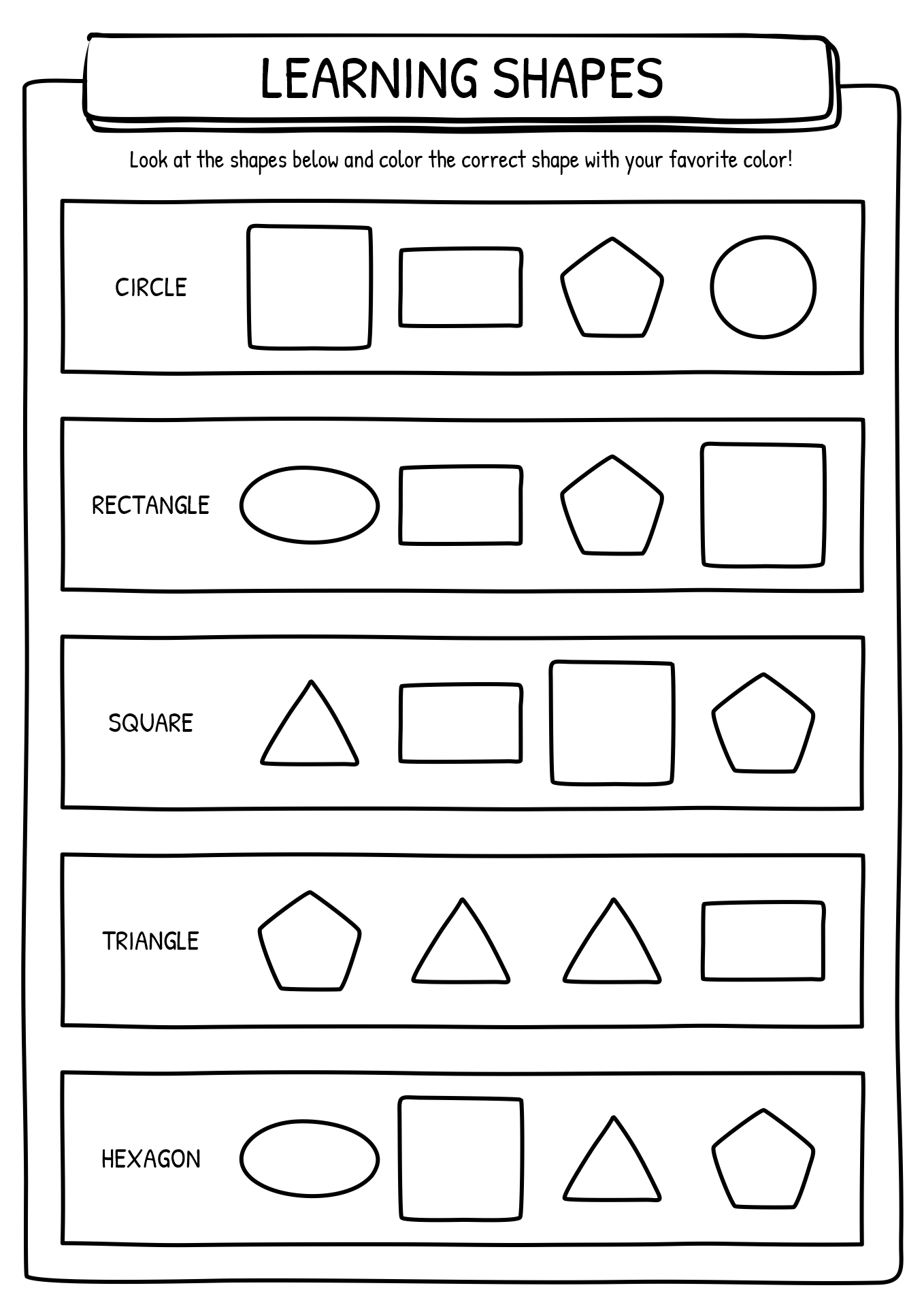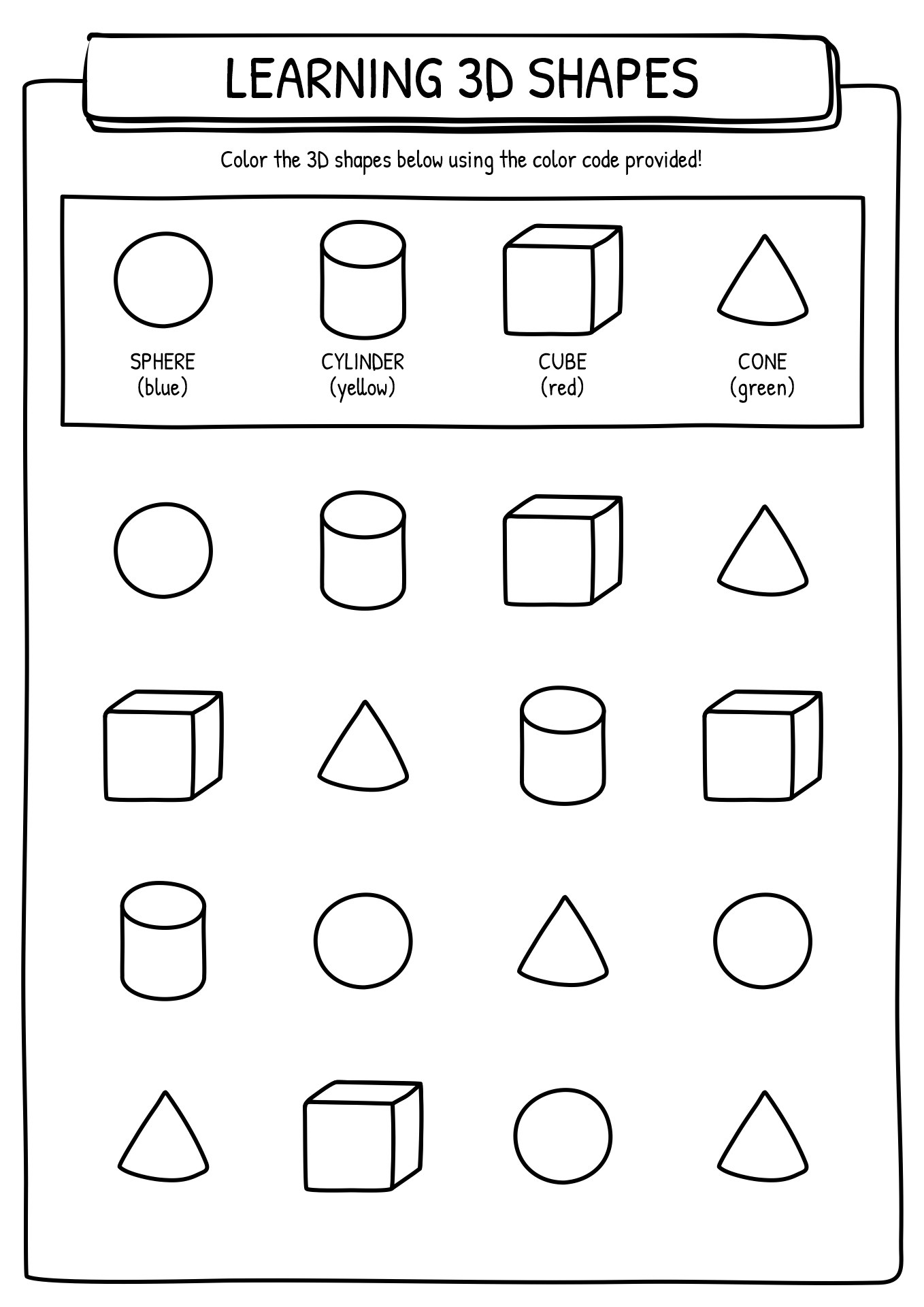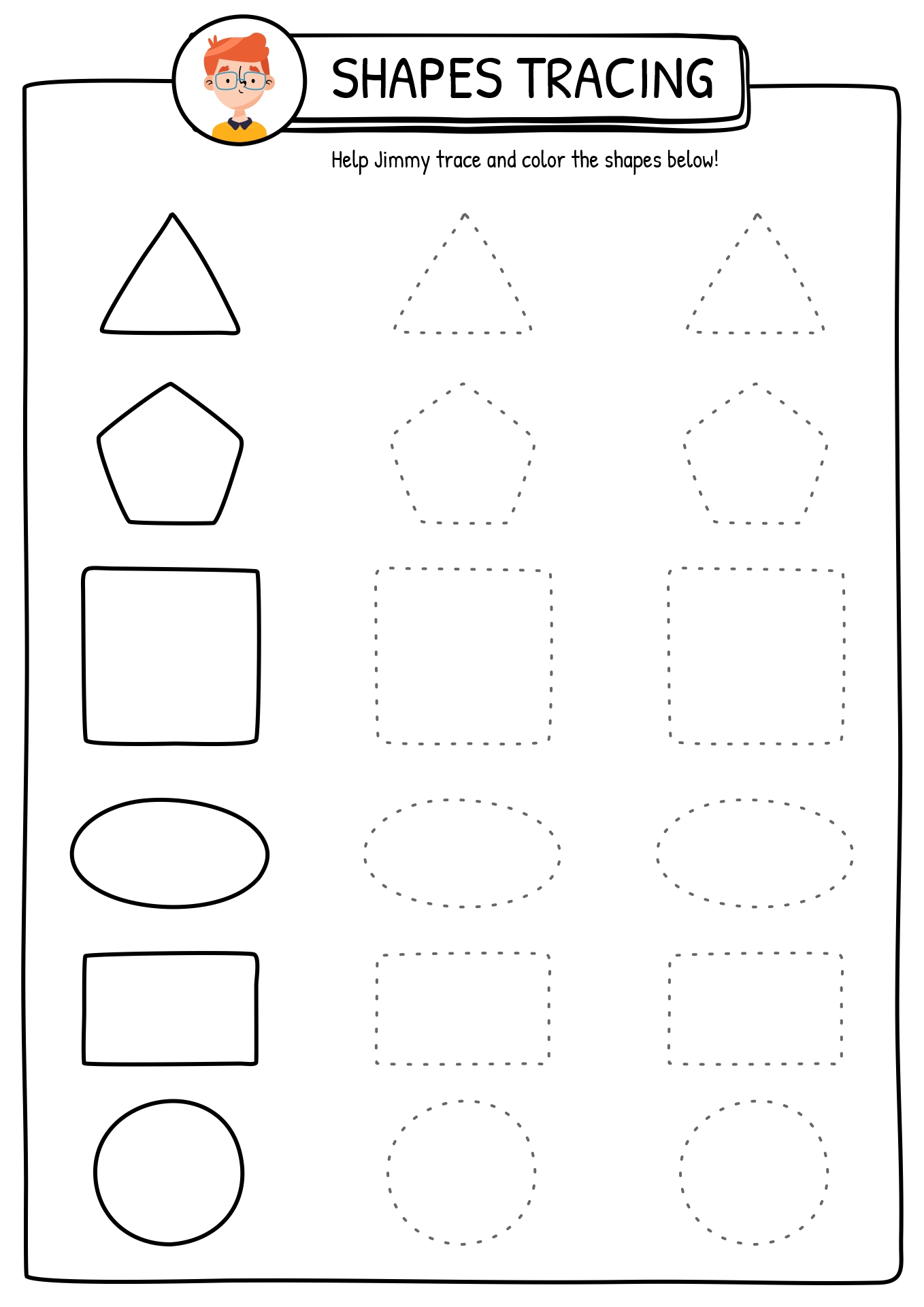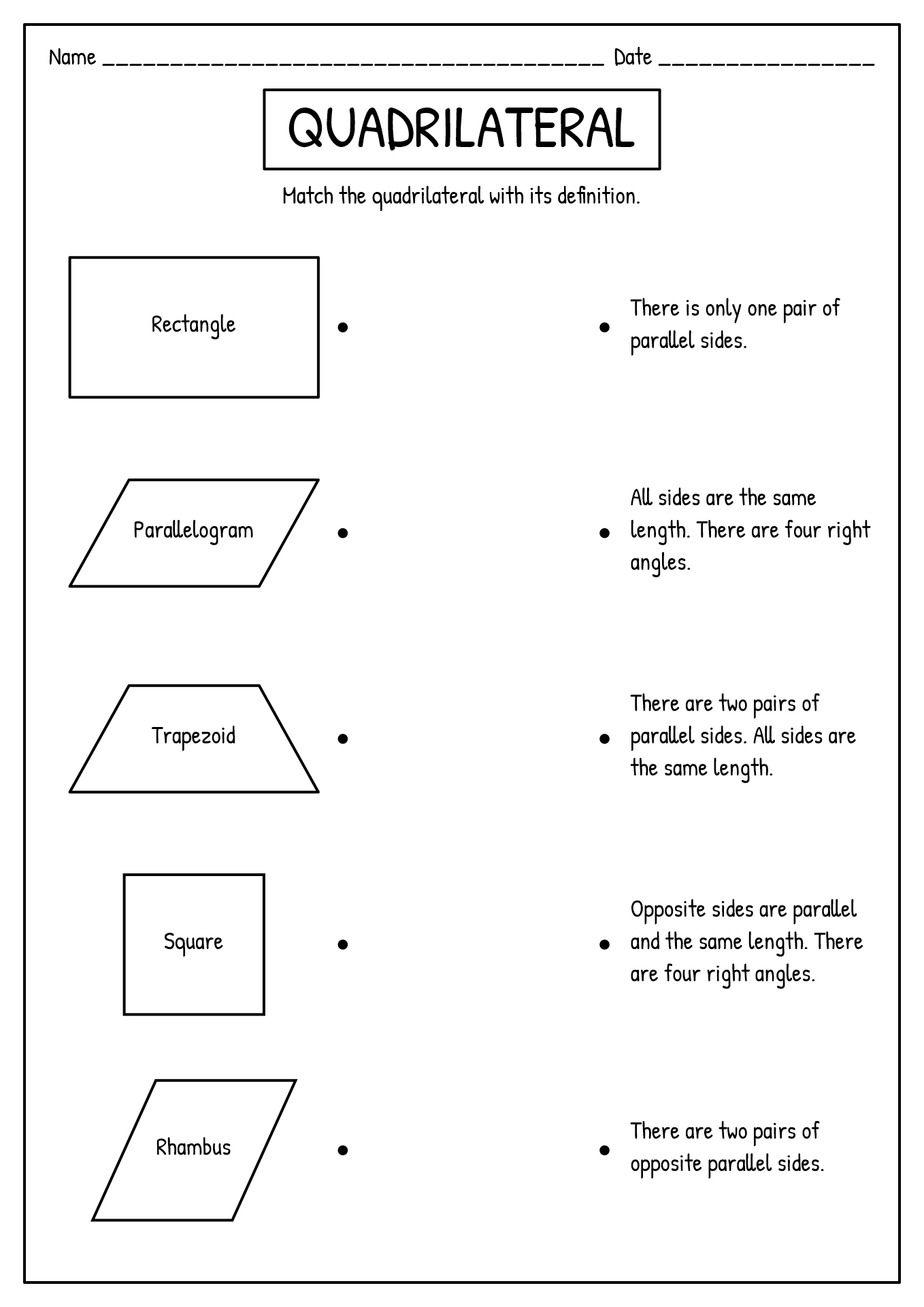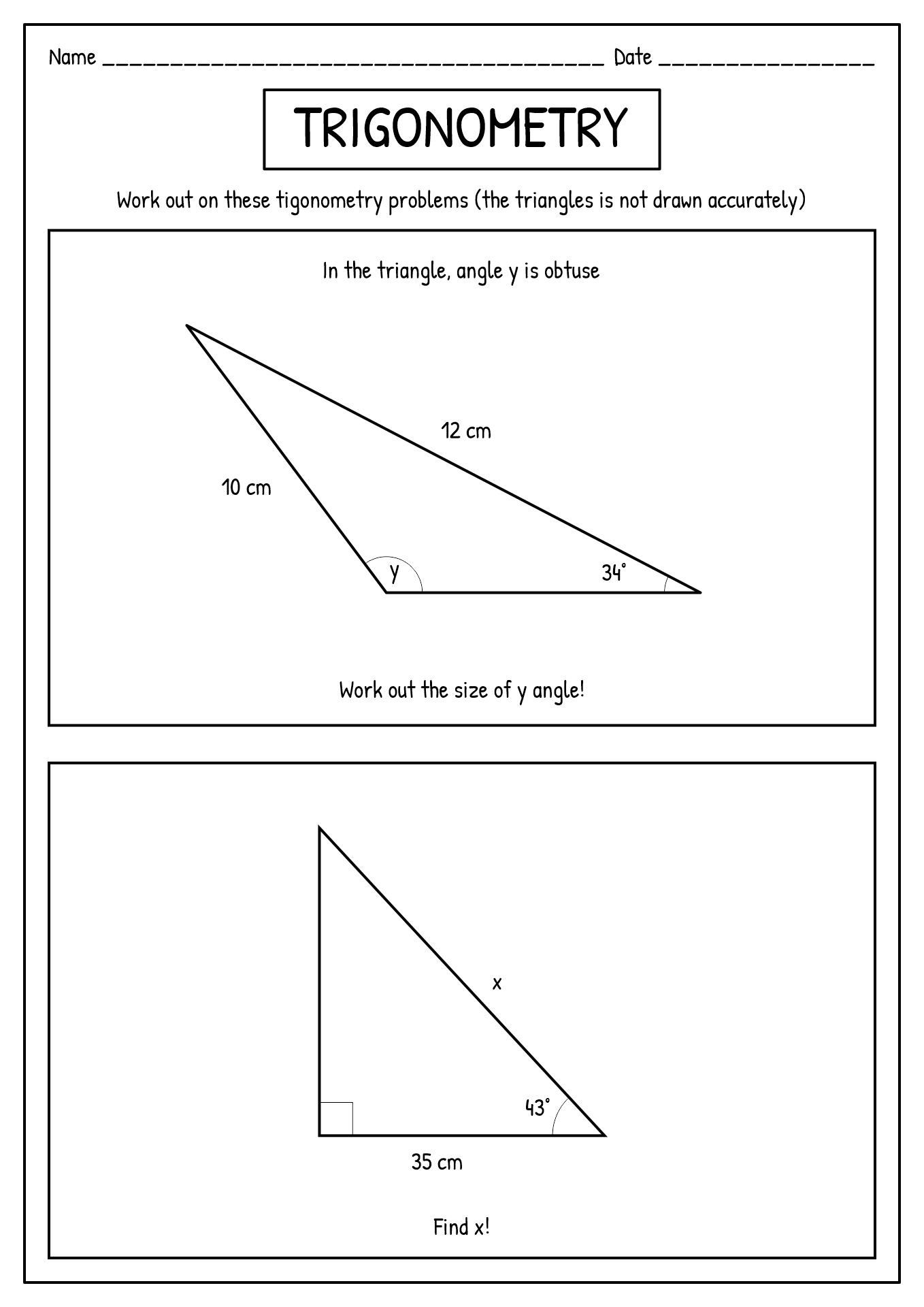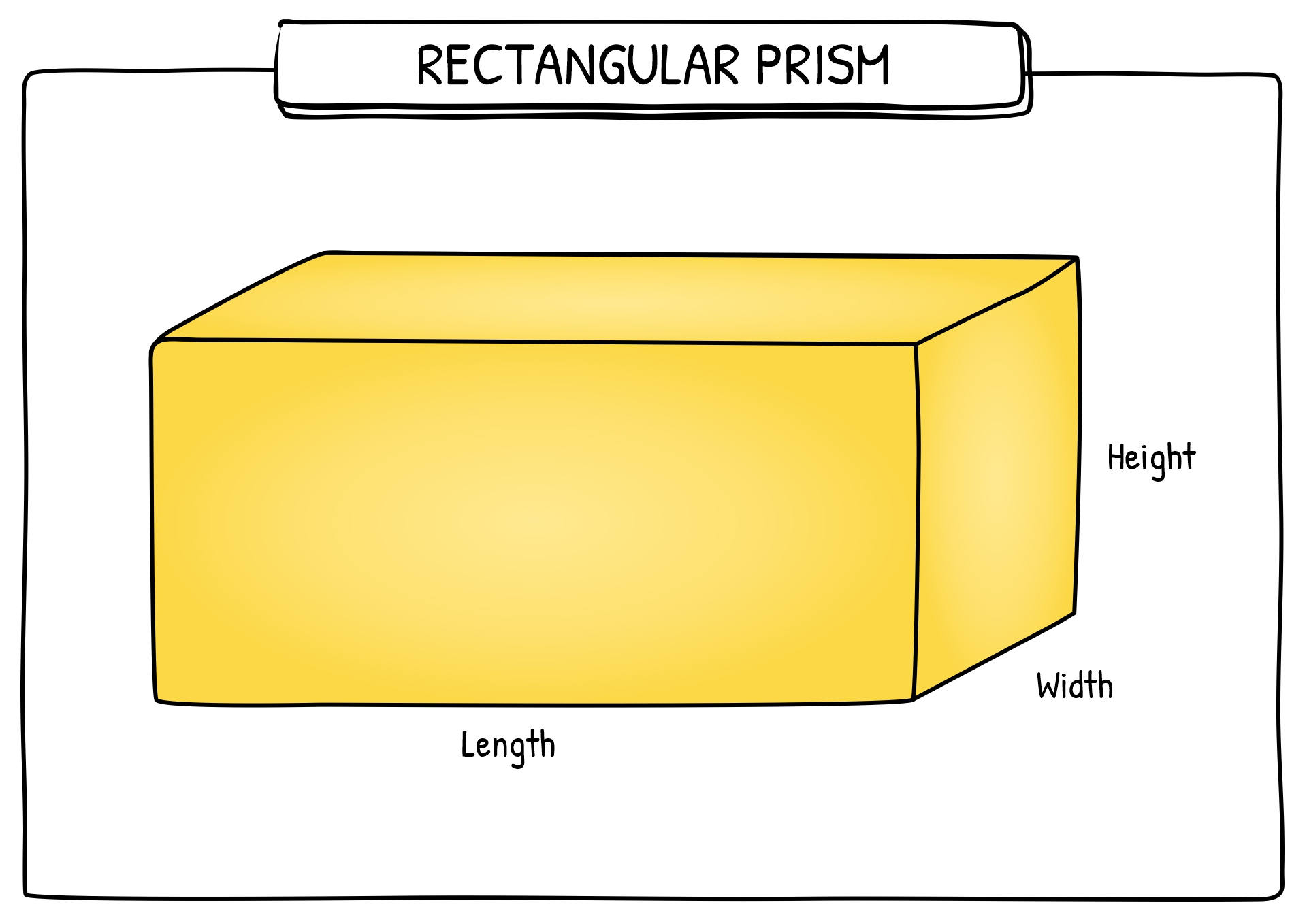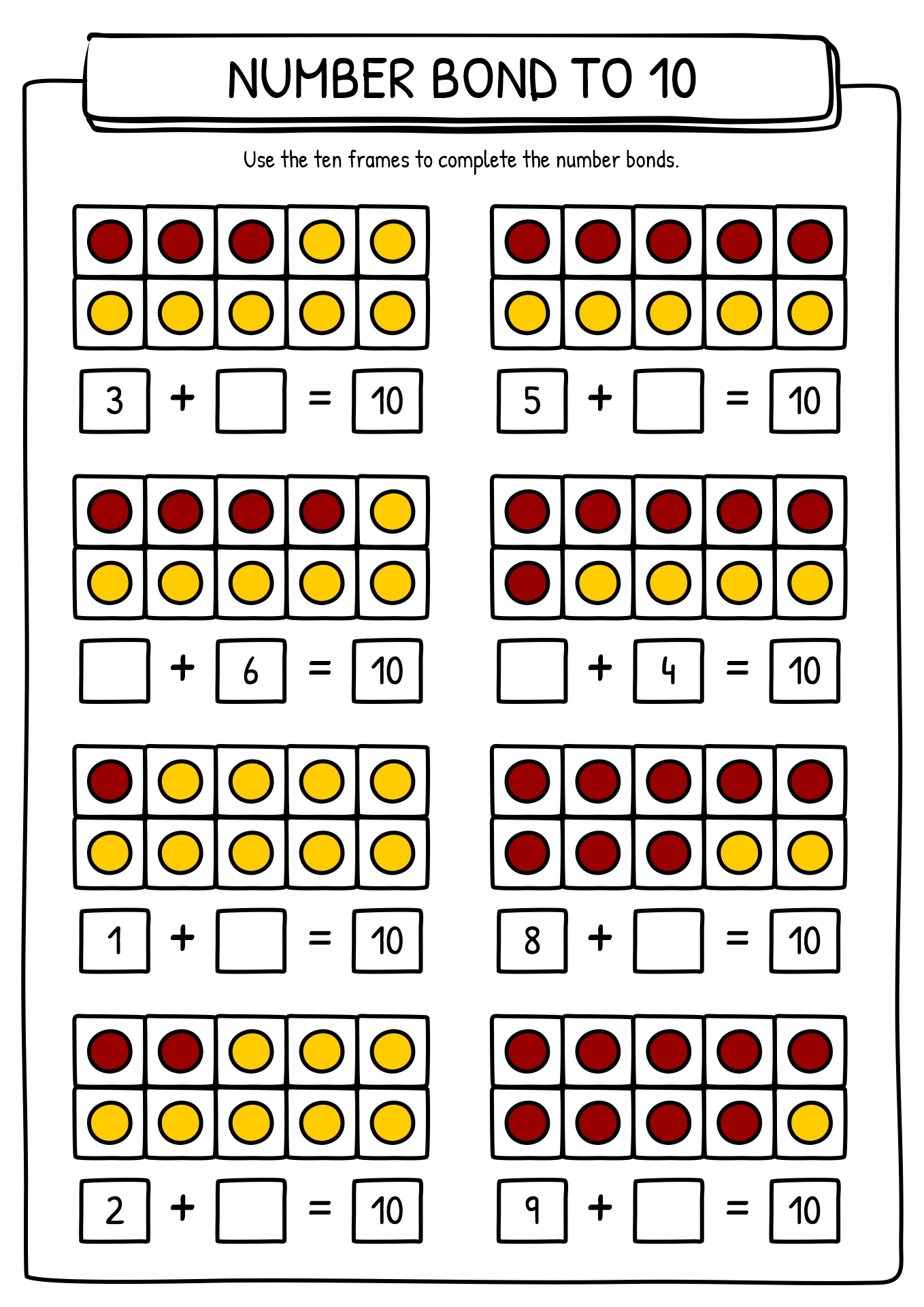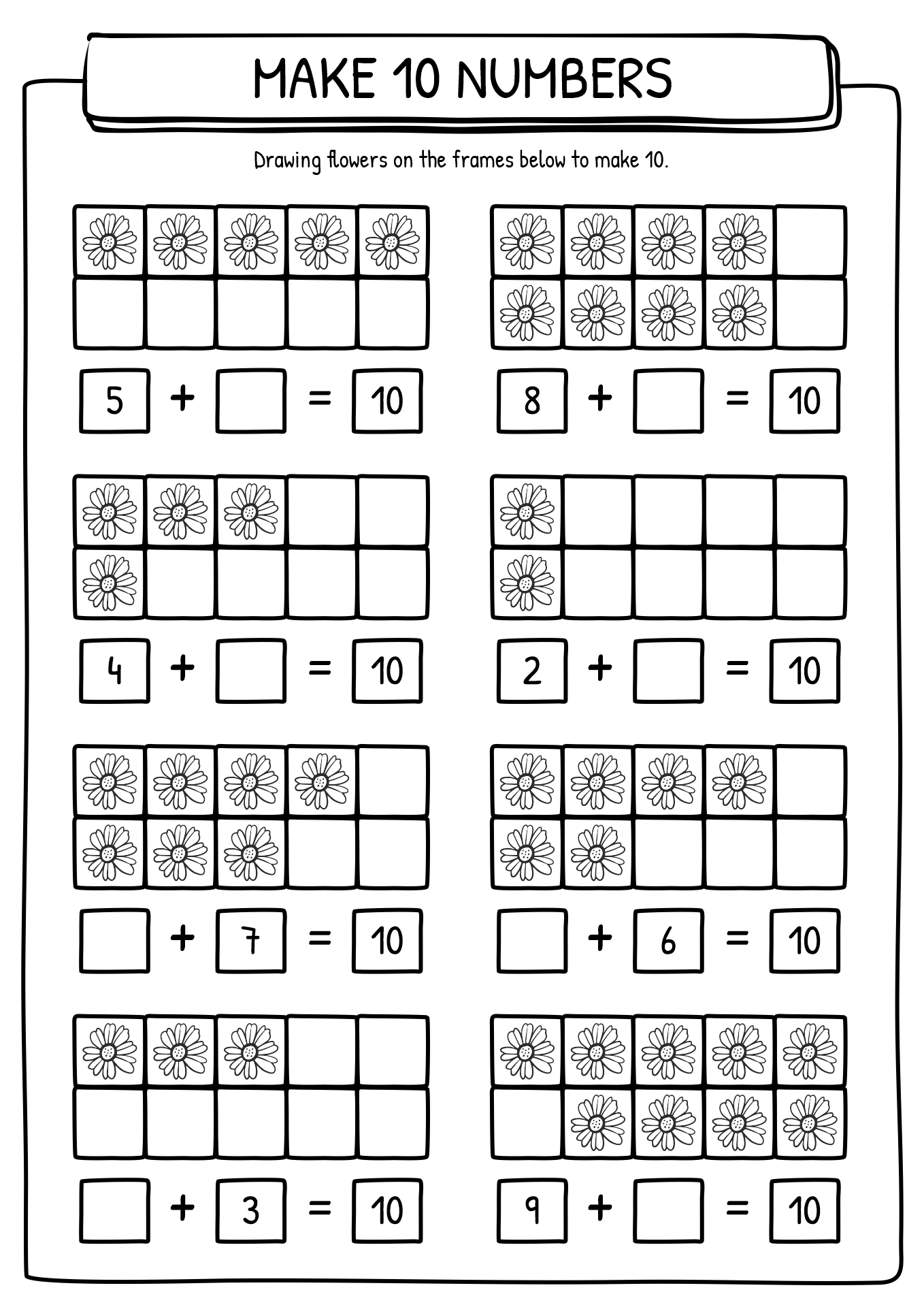Improve your spatial knowledge through these 3D Shape Properties Worksheets!

Summary: A 3D figure has three dimensions (length, depth, and width). These are forms that take up space. This feature enables us to touch and feel them. All three-dimensional shapes are dissimilar, but they share three main characteristics. Faces, edges, and vertices are the properties of 3D forms. Some education experts explained that young students' spatial skill affects their mathematical skills more than numerical ones.

### What are 3D Shapes?

Throughout primary school, young students will discover various types of 2D and 3D shapes. The letter 'D' in 2D and 3D means dimensional. Dimensional means having various features or qualities. A 2D shape is a flat form with height and width (two dimensions). Meanwhile, a 3D figure has three dimensions (length, depth, and width). These are forms that take up space. This feature enables us to touch and feel them. The main distinctness between 2D and 3D figures is their dimensions. A 2D (two-dimensional) shape has only length and height proportions. 2D shapes are planar, while 3D shapes occupy more space in three dimensions. There are various kinds of 3D forms that we could explore around us. Spheres, cubes, square pyramids, cuboids, cylinders, triangular prism, and cones are examples of 3D shapes. The children can learn with the 3D Shape Properties Worksheets to master the topic.

### What are the Properties of 3D Shapes?

All three-dimensional shapes are dissimilar, but they share three main characteristics. Faces, edges, and vertices are the properties of 3D forms. A face is a planar or curved surface of a 3D shape. Meanwhile, the edge is where two faces meet. Lastly, a vertex is a corner where edges meet. For example, a cube has eight corners, a cone has one corner, and a sphere has no corners. To understand more about this topic, young students could learn from the table below:

 Shapes Properties Sphere One face Zero vertices Zero edges Cube Six faces Eight vertices Twelve edges Square-based Pyramid Five faces Five vertices Eight edges Cuboid Six faces Eight vertices Twelve edges Cylinder Three faces Zero vertices Two edges Triangular Prism Five faces Six Vertices Nine edges Cone Two faces One vertex One edge

### Why should Children Learn 3D Shapes?

Teachers and parents should teach 3D shapes to the students and children. This knowledge is essential for the students because mastering 3D forms will help them to understand math problems better. Focusing on forms and spaces can provide an accessible route to mathematics rather than focusing primarily on numbers. It also provides opportunities for outdoor and indoor play. Some education experts explained that young students' spatial skill affects their mathematical skills more than numerical ones. Numerous studies have also shown that these skills are not innate, but teaching children spatial skills can improve math, such as number sense and general thinking. Teachers and parents can download our 3D Shapes Properties Worksheets to support young students in learning and practising.

### What are the Fun Facts about 3D Shapes?

Providing fun facts or trivia about something that you are teaching will bring extra flavour to the lesson. This trivia might excite your students and help them understand more of the context. Below are some fun facts about 3D shapes that you could share with your students:

• All 3D shapes are solids, which means you cannot have 3D shapes in different states.
• A sphere is perfectly round. Every point on every sphere's face is the same interval to the centre.
• There is a three-dimensional shape called a torus. It's exactly the shape of a doughnut.
• Egyptian pyramids are square pyramids and one of the best examples to use. Each block that makes up the pyramid weighs 2 tons.
• When you refer to something shaped like a cube, it is cubic.

A quadrilateral is a polygon shape with four precise sides. A quadrilateral could be a convex or a concave. We can classify quadrilaterals based on whether their sides, angles, diagonals, or corners have particular properties. Classifications taught in grade school include the number of parallel edge pairs, the accordance of edges, and whether all angles are right (all angles are congruent). Here are some examples of quadrilateral shapes: Kite, squares, rhombuses, rectangles, trapezoids, and parallelograms. A quadrilateral is a planar figure with four sides or edges and four corners or vertices. Corners exist at the four corners or corners of a quadrilateral. If ABCD is a quadrilateral, the vertex angles are ∠A, ∠B, ∠C, and ∠D.

A square is a closed shape, a type of polygon with four sides, four corners, and four corners. It is shaped by linking four non-collinear points. The word quadrilateral comes from the Latin words "quadra" (four) and "latus" (side). All four sides of a rectangle need not be the same length. So you can create different kinds of rectangles based on sides and angles.

The information, names, images and video detail mentioned are the property of their respective owners & source.

### Popular Categories

Have something to tell us about the gallery?

Submit